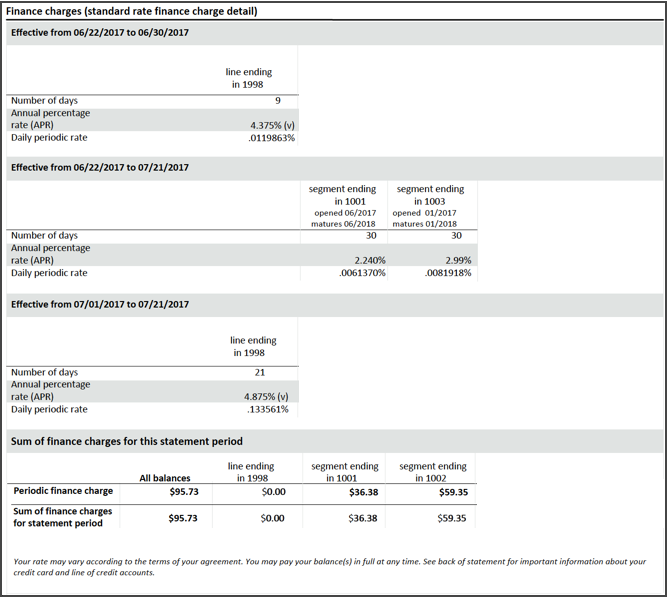Navegó a una página que no está disponible en español en este momento. Seleccione el enlace si desea ver otro contenido en español.

Página principal

# Finance Charges

The Finance charges section of your statement provides information about how we calculate the finance charges on your account, including the individual segments, if applicable. This area of the statement will also include the annual percentage rate, periodic rate, and the number of days the rates are effective.## Segment

A segment represents a portion of the balance of your account either in a fixed or variable rate pricing. Also referred to as: FRA (fixed-rate advance), FRO (fixed-rate option), RLO (rate lock option), or variable/promo.

## Annual percentage rate

The cost to borrow money expressed as a yearly percentage.

## Daily periodic rate

A type of interest/finance charge calculation. A daily periodic interest rate is calculated by dividing the annual percentage rate (APR) by either 360, 365, or 366 days, depending on the account agreement. The resulting daily periodic interest rate is then used to calculate interest by multiplying the rate by the applicable balance and number of days in the billing cycle.